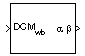# Direction Cosine Matrix Body to Wind to Alpha and Beta

Convert direction cosine matrix to angle of attack and sideslip angle

• Library:
• Aerospace Blockset / Utilities / Axes Transformations

•## Description

The Direction Cosine Matrix Body to Wind to Alpha and Beta block converts a 3-by-3 direction cosine matrix (DCM) to angle of attack and sideslip angle. The DCM performs the coordinate transformation of a vector in body axes (ox0, oy0, oz0) into a vector in wind axes (ox2, oy2, oz2). For more information on the direction cosine matrix, see Algorithms.

## Limitations

• This implementation generates angles that lie between ±90 degrees.

## Ports

### Input

expand all

Direction cosine matrix to transform body-fixed vectors to wind-fixed vectors, specified as a 3-by-3 direct cosine matrix.

Data Types: `double`

### Output

expand all

Angle of attack and sideslip angle, returned as a vector, in radians.

Data Types: `double`

## Parameters

expand all

Block behavior when the direction cosine matrix is invalid (not orthogonal).

• `Warning` — Displays warning indicating that the direction cosine matrix is invalid.

• `Error` — Displays error indicating that the direction cosine matrix is invalid.

• `None` — Does not display warning or error (default).

#### Programmatic Use

 Block Parameter: `action` Type: character vector Values: `'None'` | `'Warning'` | `'Error'` Default: `'None'`

Data Types: `char` | `string`

Tolerance of the direction cosine matrix validity, specified as a scalar. The block considers the direction cosine matrix valid if these conditions are true:

• The transpose of the direction cosine matrix times itself equals `1` within the specified tolerance (`transpose(n)*n == 1±tolerance`).

• The determinant of the direction cosine matrix equals `1` within the specified tolerance (`det(n) == 1±tolerance`).

#### Programmatic Use

 Block Parameter: `tolerance` Type: character vector Values: `'eps(2)'` | scalar Default: `'eps(2)'`

Data Types: `double`

## Algorithms

The DCM matrix performs the coordinate transformation of a vector in body axes (ox0, oy0, oz0) into a vector in wind axes (ox2, oy2, oz2). The order of the axis rotations required to bring this about is:

1. A rotation about oy0 through the angle of attack (α) to axes (ox1, oy1, oz1)

2. A rotation about oz1 through the sideslip angle (β) to axes (ox2, oy2, oz2)

`$\begin{array}{l}\left[\begin{array}{c}o{x}_{2}\\ o{y}_{2}\\ o{z}_{2}\end{array}\right]=DC{M}_{wb}\left[\begin{array}{c}o{x}_{0}\\ o{y}_{0}\\ o{z}_{0}\end{array}\right]\\ \\ \left[\begin{array}{c}o{x}_{2}\\ o{y}_{2}\\ o{z}_{2}\end{array}\right]=\left[\begin{array}{ccc}\mathrm{cos}\beta & \mathrm{sin}\beta & 0\\ -\mathrm{sin}\beta & \mathrm{cos}\beta & 0\\ 0& 0& 1\end{array}\right]\left[\begin{array}{ccc}\mathrm{cos}\alpha & 0& \mathrm{sin}\alpha \\ 0& 1& 0\\ -\mathrm{sin}\alpha & 0& \mathrm{cos}\alpha \end{array}\right]\left[\begin{array}{c}o{x}_{0}\\ o{y}_{0}\\ o{z}_{0}\end{array}\right]\end{array}$`

Combining the two axis transformation matrices defines the following DCM.

`$DC{M}_{wb}=\left[\begin{array}{ccc}\mathrm{cos}\alpha \mathrm{cos}\beta & \mathrm{sin}\beta & \mathrm{sin}\alpha \mathrm{cos}\beta \\ -\mathrm{cos}\alpha \mathrm{sin}\beta & \mathrm{cos}\beta & -\mathrm{sin}\alpha \mathrm{sin}\beta \\ -\mathrm{sin}\alpha & 0& \mathrm{cos}\alpha \end{array}\right]$`

To determine angles from the DCM, the following equations are used:

`$\begin{array}{l}\alpha =\text{asin}\left(-DCM\left(3,1\right)\right)\\ \\ \beta =\text{asin}\left(DCM\left(1,2\right)\right)\end{array}$`

 Stevens, Brian L., Frank L. Lewis. Aircraft Control and Simulation, Second Edition. Hoboken, NJ: Wiley–Interscience.

## Support평가판 신청